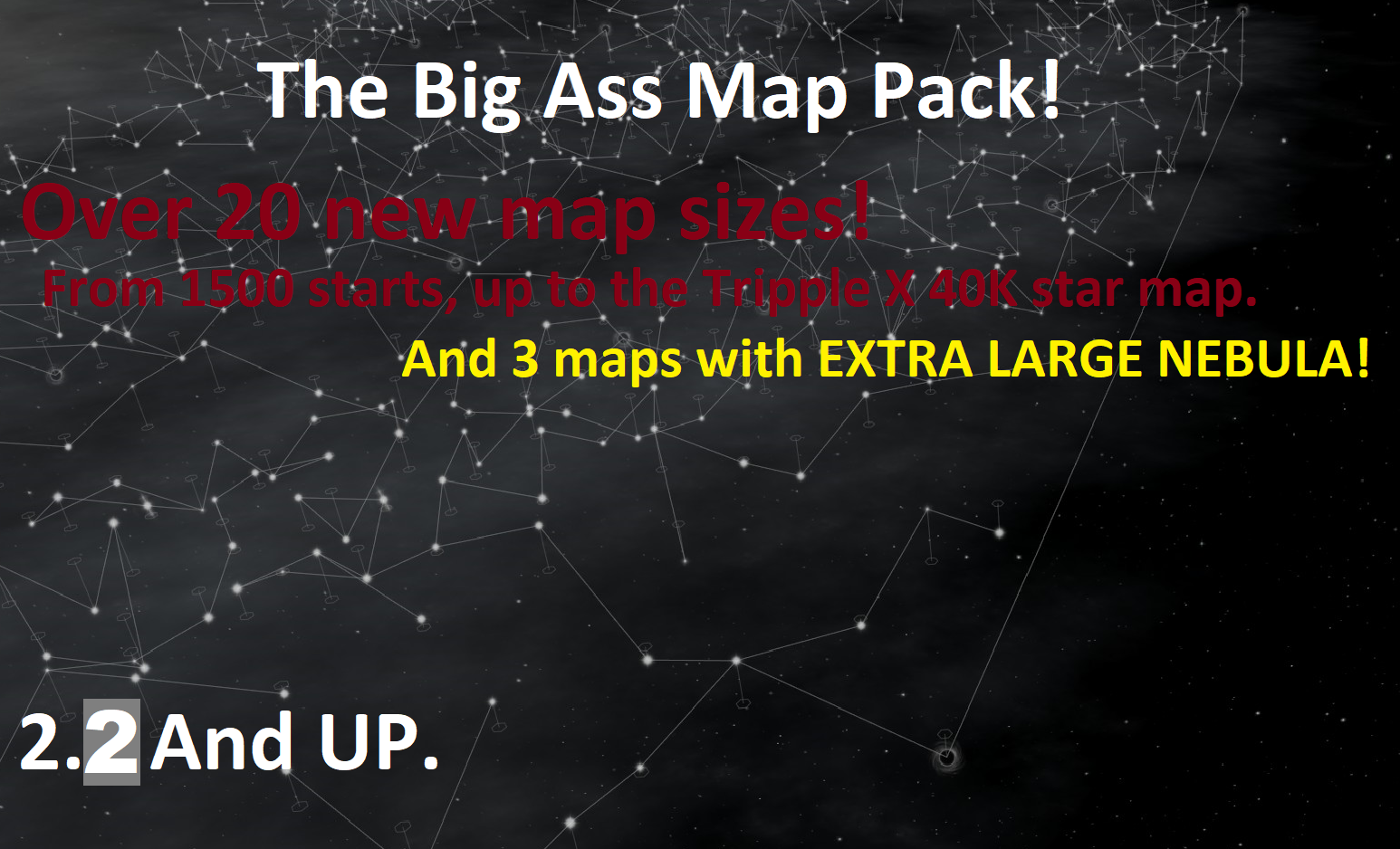The Jagji’s Big Ass Maps Pack

If you liked this item, please rate it up on Steam Workshop page.Author: TheJagji

Last revision: 6 Dec, 2018 at 21:51 UTC (1)

File size: 0 B

On Steam Workshop

Description:

—– This is the 2.2 ——-
For a 1.9 of this mod, look HERE
***Name List Mod Is REQUIRED***
***If you like it, please rate it. 🙂 ***

______________________________________________________

So, what dose this mod include?

New galaxy sizes. 22 of them.

More Wormholes and Gates for the bigger maps, less on the single small map.

Bit of info on the following maps:

> S = Stars
> WP = Wormhole Pairs
> GN = Gate Number
> EC = Empire Count

MAP LIST

"Micro" – S=150 WP=1, GN=3, FE=2, M=2
Perfict for a 1v1 with a frined, or AI.

"Enormous" – S=1500, WP=5, GN=5, EC=25
"Jumbo" – S=2000, WP=5, GN=5, EC=30
"Substantial" – S=2500, WP=5, GN=5, EC=30
"Massive" – S=3000, WP=6, GN=6, EC=30
"Bulky" – S=3500, WP=6, GN=6, EC=30
"Mammoth" – S=4000, WP=6, GN=6, EC=30
"Gargantuan" – S=4500, WP=7, GN=7, EC=30
"Walloping" – S=5000, WP=7, GN=7, EC=30
"Gigantic" – S=5500, WP=7, GN=7, EC=30
"Awesome" – S=6000, WP=8, GN=8, EC=30
"Copious" – S=6500, WP=8, GN=8, EC=30
"Tremendous" – S=7000, WP=8, GN=8, EC=30
"Hulking" – S=7500, WP=9, GN=9, EC=30
"Alarming" – S=8000, WP=9, GN=9, EC=30
"Vast" – S=8500, WP=9, GN=9, EC=30
"Superabundant" – S=9000, WP=10, GN=10, EC=35
"Overflowing" – S=9500, WP=10, GN=10, EC=35
"Brobdingnagian" – S=10000, WP=10, GN=10, EC=40
"X" – S=20000, WP=50, GN=50, EC=100
"XX" – S=30000, WP=100, GN=100, EC=100 (may not work, use at your own risk)
"XXX" – S=40000, WP=100, GN=100, EC=100 (may not work, use at your own risk)

Nebular Maps: The following maps are desinded to try and cover the whole map in nebular.

> S = Stars
> NN = Nebular Number
> NS = Nebular Size
> ND = Nebular Min Distence

"Adventure" – S=1000, WP=6, GN=6, EC=20, NN=20, NS=100, ND=100
"Expedition" – S=2000, WP=5, GN=5, EC=30, NN=25, NS=100, ND=100
"The Quest" – S=3000, WP=6, GN=6, EC=30, NN=30, NS=100, ND=100